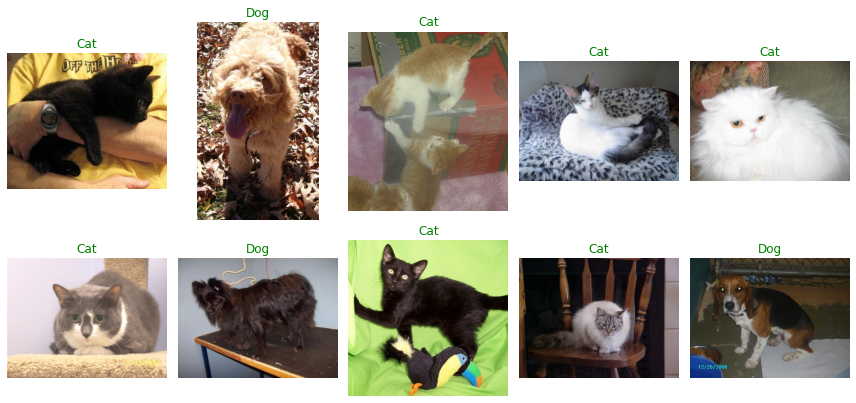# PyTorch and Albumentations for image classification¶

This example shows how to use Albumentations for image classification. We will use the `Cats vs. Docs` dataset. The task will be to detect whether an image contains a cat or a dog.

### Import the required libraries¶

``````from collections import defaultdict
import copy
import random
import os
import shutil
from urllib.request import urlretrieve

import albumentations as A
from albumentations.pytorch import ToTensorV2
import cv2
import matplotlib.pyplot as plt
from tqdm import tqdm
import torch
import torch.backends.cudnn as cudnn
import torch.nn as nn
import torch.optim
import torchvision.models as models

cudnn.benchmark = True
``````

``````class TqdmUpTo(tqdm):
def update_to(self, b=1, bsize=1, tsize=None):
if tsize is not None:
self.total = tsize
self.update(b * bsize - self.n)

directory = os.path.dirname(os.path.abspath(filepath))
os.makedirs(directory, exist_ok=True)
if os.path.exists(filepath):
return

with TqdmUpTo(unit="B", unit_scale=True, unit_divisor=1024, miniters=1, desc=os.path.basename(filepath)) as t:
urlretrieve(url, filename=filepath, reporthook=t.update_to, data=None)
t.total = t.n

def extract_archive(filepath):
extract_dir = os.path.dirname(os.path.abspath(filepath))
shutil.unpack_archive(filepath, extract_dir)
``````

``````dataset_directory = os.path.join(os.environ["HOME"], "datasets/cats-vs-dogs")
``````

### Download and extract the `Cats vs. Docs` dataset¶

``````filepath = os.path.join(dataset_directory, "kagglecatsanddogs_3367a.zip")
filepath=filepath,
)
extract_archive(filepath)
``````
``````Filepath already exists. Skipping download.
```
```

### Split files from the dataset into the train and validation sets¶

Some files in the dataset are broken, so we will use only those image files that OpenCV could load correctly. We will use 20000 images for training, 4936 images for validation, and 10 images for testing.

``````root_directory = os.path.join(dataset_directory, "PetImages")

cat_directory = os.path.join(root_directory, "Cat")
dog_directory = os.path.join(root_directory, "Dog")

cat_images_filepaths = sorted([os.path.join(cat_directory, f) for f in os.listdir(cat_directory)])
dog_images_filepaths = sorted([os.path.join(dog_directory, f) for f in os.listdir(dog_directory)])
images_filepaths = [*cat_images_filepaths, *dog_images_filepaths]
correct_images_filepaths = [i for i in images_filepaths if cv2.imread(i) is not None]

random.seed(42)
random.shuffle(correct_images_filepaths)
train_images_filepaths = correct_images_filepaths[:20000]
val_images_filepaths = correct_images_filepaths[20000:-10]
test_images_filepaths = correct_images_filepaths[-10:]
print(len(train_images_filepaths), len(val_images_filepaths), len(test_images_filepaths))
``````
``````20000 4936 10
```
```

### Define a function to visualize images and their labels¶

Let's define a function that will take a list of images' file paths and their labels and visualize them in a grid. Correct labels are colored green, and incorrectly predicted labels are colored red.

``````def display_image_grid(images_filepaths, predicted_labels=(), cols=5):
rows = len(images_filepaths) // cols
figure, ax = plt.subplots(nrows=rows, ncols=cols, figsize=(12, 6))
for i, image_filepath in enumerate(images_filepaths):
image = cv2.cvtColor(image, cv2.COLOR_BGR2RGB)
true_label = os.path.normpath(image_filepath).split(os.sep)[-2]
predicted_label = predicted_labels[i] if predicted_labels else true_label
color = "green" if true_label == predicted_label else "red"
ax.ravel()[i].imshow(image)
ax.ravel()[i].set_title(predicted_label, color=color)
ax.ravel()[i].set_axis_off()
plt.tight_layout()
plt.show()
``````
``````display_image_grid(test_images_filepaths)
``````### Define a PyTorch dataset class¶

Next, we define a PyTorch dataset. If you are new to PyTorch datasets, please refer to this tutorial - https://pytorch.org/tutorials/beginner/data_loading_tutorial.html.

Out task is binary classification - a model needs to predict whether an image contains a cat or a dog. Our labels will mark the probability that an image contains a cat. So the correct label for an image with a cat will be `1.0`, and the correct label for an image with a dog will be `0.0`.

`__init__` will receive an optional `transform` argument. It is a transformation function of the Albumentations augmentation pipeline. Then in `__getitem__`, the Dataset class will use that function to augment an image and return it along with the correct label.

``````class CatsVsDogsDataset(Dataset):
def __init__(self, images_filepaths, transform=None):
self.images_filepaths = images_filepaths
self.transform = transform

def __len__(self):
return len(self.images_filepaths)

def __getitem__(self, idx):
image_filepath = self.images_filepaths[idx]
image = cv2.cvtColor(image, cv2.COLOR_BGR2RGB)
if os.path.normpath(image_filepath).split(os.sep)[-2] == "Cat":
label = 1.0
else:
label = 0.0
if self.transform is not None:
image = self.transform(image=image)["image"]
return image, label
``````

### Use Albumentations to define transformation functions for the train and validation datasets¶

We use Albumentations to define augmentation pipelines for training and validation datasets. In both pipelines, we first resize an input image, so its smallest size is 160px, then we take a 128px by 128px crop. For the training dataset, we also apply more augmentations to that crop. Next, we will normalize an image. We first divide all pixel values of an image by 255, so each pixel's value will lie in a range `[0.0, 1.0]`. Then we will subtract mean pixel values and divide values by the standard deviation. `mean` and `std` in augmentation pipelines are taken from the `ImageNet` dataset. Still, they transfer reasonably well to the `Cats vs. Dogs` dataset. After that, we will apply `ToTensorV2` that converts a NumPy array to a PyTorch tensor, which will serve as an input to a neural network.

Note that in the validation pipeline we will use `A.CenterCrop` instead of `A.RandomCrop` because we want out validation results to be deterministic (so that they will not depend upon a random location of a crop).

``````train_transform = A.Compose(
[
A.SmallestMaxSize(max_size=160),
A.ShiftScaleRotate(shift_limit=0.05, scale_limit=0.05, rotate_limit=15, p=0.5),
A.RandomCrop(height=128, width=128),
A.RGBShift(r_shift_limit=15, g_shift_limit=15, b_shift_limit=15, p=0.5),
A.RandomBrightnessContrast(p=0.5),
A.Normalize(mean=(0.485, 0.456, 0.406), std=(0.229, 0.224, 0.225)),
ToTensorV2(),
]
)
train_dataset = CatsVsDogsDataset(images_filepaths=train_images_filepaths, transform=train_transform)
``````
``````val_transform = A.Compose(
[
A.SmallestMaxSize(max_size=160),
A.CenterCrop(height=128, width=128),
A.Normalize(mean=(0.485, 0.456, 0.406), std=(0.229, 0.224, 0.225)),
ToTensorV2(),
]
)
val_dataset = CatsVsDogsDataset(images_filepaths=val_images_filepaths, transform=val_transform)
``````

Also let's define a function that takes a dataset and visualizes different augmentations applied to the same image.

``````def visualize_augmentations(dataset, idx=0, samples=10, cols=5):
dataset = copy.deepcopy(dataset)
dataset.transform = A.Compose([t for t in dataset.transform if not isinstance(t, (A.Normalize, ToTensorV2))])
rows = samples // cols
figure, ax = plt.subplots(nrows=rows, ncols=cols, figsize=(12, 6))
for i in range(samples):
image, _ = dataset[idx]
ax.ravel()[i].imshow(image)
ax.ravel()[i].set_axis_off()
plt.tight_layout()
plt.show()
``````
``````random.seed(42)
visualize_augmentations(train_dataset)
``````